# Current for Realtime Machine Learning

Since 2014, I am working on Current: The C++ framework for realtime machine learning.

Realtime adaptive behavior is the theme for backends these days. With Current, we make it easy to add AI capabilities to existing APIs. Training and applying models in early 21st century should be as simple as sending and receiving HTTP headers is since late 20th.

Any bit of data is something machine learning models will need for realtime decision making. This philosophy is the cornerstone of Current. As time went by, it became clear this vision has been the right one from day one.

To enable this on the infrastructure level, every piece of data belongs to a strongly-typed immutable event log. This event log is the single source of truth for both the event itself and the domain it contributes to.

The above approach scales naturally to the application-wide persistent publish-subscribe bus. Materialized views emerge where structured tables used to be. Isolation primitives are transactions, which in Current are native lambda functions. Following the actor model design, transactions can be replayed as part of stream replication. Atop this, we added out-of-the-box RESTful APIs, CQS support, and a convex optimization engine that uses the same code to train and apply models.

Natively, Current produces binaries that can hold thousands of HTTP QPS end-to-end off a single CPU. If someone asks whether your binary is a database or an application server, the answer will be an unambiguous “Yes”.

Think of a strongly-typed Kafka and Redis running as part of your code, add zero-downtime follower-to-master flip capabilities, blend in per-query model application, and, chances are, you’ll imagine almost exactly what Current had implemented and battle-tested as of a year ago.

Running Current in production for over a year, we have strengthened our belief the MVP we built is exactly the convex hull of what it takes to prove Current’s mission. Today, we are standing on the shoulder of this giant: the framework makes bringing machine learning into application APIs as simple it is today to configure Jenkins, plug in a Slack bot, or enabling web analytics reporting.

Our approach to realtime machine learning deserves more attention, and that’s what this post is about. While designing the system from scratch in C++ is a paradigm shift for many, training and applying models is the piece that can be used out of the box by any junior C++ developer, with no commitment to ship Current to the backend stack, and, I keep my fingers crossed, without ever having to reach out to us for help.

Credentials first. I am a data engineer. Software engineering and data infrastructure are my strengths; my passion, however, is working with models, sometimes training dozens a day, validating them offline and online, and, ultimately, shipping the best ones.

To summarize my broad experience, the large and yet unbridged gap in machine learning is the language barrier between data scientists and software engineers. Yes, the one you’ll hear of on any data science meetup. That’s exactly the field where I’m making a living for the past several years.

Data scientists are proficient at understanding the underlying data and building models atop it. And, way more often than not, we build models using the tools that do not map well to what can be launched in production. More importantly, a lion share of the work of the data scientist is massaging the data, cleaning up the features, creating the new ones, sometimes pruning rows or columns, normalizing and calibrating input, etc. Needless to say, the result of the above, while often impressive when presented on a big screen from a PowerPoint slide, is generally not ready to be shipped live, at least by the standards developers would require it to be.

Engineers, and, especially, DevOps, look at the result of the data scientists’ work from a different perspective. First, speaking of realtime machine learning, we need the model to be applied at the query time. Which means along with the carefully trained model parameters vector we need the piece of code to apply this model; the code that’s both performant to serve the traffic and robust to not crash if some division by zero or a square root of a negative occurs. Then, the model should cover all possible input cases: including the rare ones data scientists have dismissed as noise, and including the yet unseen ones as long as they fit the broader set of constraints assumed. On top of the above, we need monitoring tools: if suddenly our model misbehaves, we need to know about it ASAP. Last, but not least: we need a big red button, a downgrade switch to quickly revert back to the old model, be it just the parameters vector, or a different piece of code altogether.

Sometimes a requirement as simple as “a percentile feature” is the blocker; in a good organization where scientists work closely with engineers it would be caught quickly. Sometimes that one “not-too-but-still-quite important” feature is taken from a different data source, making it impossible to access directly at the query time. Sometimes we discover the model doesn’t perform as well because somehow what should only have been part of the test set has penetrated into the training one.

A large part of our motivation with Current has been to make sure data scientists and engineers speak the same language.

To accomplish this goal, this language is C++. Don’t panic, it’s a very small subset of the language, which reads just like JavaScript or Objective C. And even if the only language you speak is Python, x * (1.0 / (1.0 + exp(-x)) can hardly be unintuitive.

We get a huge win right away as the result of this move: the code to compute features on the training data during the manual, batch, training phase, is the same code that runs in production at thousands of queries per second. The very semantics of the process ensures the code a data scientist writes is by itself shippable.

Not a bit less importantly, as we can use the whole power of C++, Current includes the complete implementation of the module to perform analytical differentiation as part of the convex optimization engine. In plain English it means: as long as you can make your cost function relatively low-parametric and relatively convex, you only need to implement this very function (well, and define the starting point), and the machine will do everything else for you.

For the demo, let’s take a look at the Iris Flowers dataset. Among many reasons to choose it are:

• It’s low-dimensional: just four variables makes the data easy to visualize.
• It is dense: there are no missing values to take care of.
• It is calibrated: possible laymen assumptions about what Euclidian distance could mean will pass the reality check.
• It is labeled: classification problems are perfect for simple demonstrations.
• It is balanced: of three classes, there are exactly 50 examples of each.

Nuff said. Here is the data plotted by the Current-based code itself:

First, let’s train a descriptive model finding an ellipsoid (with axes parallel to the four dimensions) that best captures each of three classes. Yes, there is a closed-form solution for this, but look now neat the cost function and the optimization code is. Here’s the 4D visualization of the above:

Ultimately, in the real world, we want to know how well the model performs the task of telling cases of one class from the cases of another. To visualize this, let’s examine the histogram of scores. The model, the descriptive one so far, outputs the probability of whether an example of class X is predicted to belong to class Y. For X == Y, the predicted class being the true class, we would expect to see the probabilities equal or very close to one; similarly, for X != Y the resulting probabilities should be zero or very close to zero.

Three possible values for X and Y make for a good 3x3 matrix of histograms. Model outputs are probabilities, so each histogram plots the frequencies of values from zero to one. Rows are the true labels of the cases/flowers, and the columns are the models applied. These two pieces of code produce the following output:

In the perfect world, on the main diagonal there would be three histograms which only output the scores of 1.0, a single bar on the very right. The other six histograms, in this perfect world, would show all 0.0, a single bar on the very left.

The world we live in is perfect for Setosa, the top row and the leftmost column. The Setosa flowers are easy to tell from the others. Among the other two, however, the Versicolor ones and the Virginica ones, there is indeed some room for confusion, which accidental small bars here and there show. Note how quite a few non-perfect scores are present in either direction (false positives and false negatives), and the absolute values of sub-perfect model outputs span the whole probabilities range.

In fact, when evaluating the descriptive model by the classification accuracy metric, its performance is 0.84. This is the value of the log loss objective, normalized back as the probability.

or the readers who are not data scientists:

• There are three ellipsoids, one per class.
• Each ellipsoid is treated as a multidimensional Gaussian, with the axes parallel to the coordinate axes, for the sake of simplicity.

• The “coordinates” of this datapoint, “x”, the four-dimensional vector, are fed into the three Gaussians. If classes are A, B, and C, the three outputs are f_A(x), f_B(x), and f_C(x).
• All three are non-negative, as they are the the values of the probability density function.
• The model output, which is the final probability, is assumed to be f_T(x) / (f_A(x) + f_B(x) + f_C(x)), where T is the “truth”: the class to which this datapoint does belong.
• Note that this way the output for each datapoint is by definition a three-dimensional simplex; a probability distribution over the three classes, or, in other words, three non-negative numbers that sum up to one.
• Practically speaking, as Gaussians fade away quickly, for many points in our space the output would be very close to 1.0, as two of the three values of f_X() will be almost indistinguishable from zero.
• The log-loss cost function is the sum of the logarithms of model outputs for the “true” class across all the points. The loss is to be minimized.

To normalize this cost function to a human-readable number, note that:

• The number of datapoints is a constant, so optimizing the sum of the logarithms is the same as optimizing the average value of the logarithm.
• The exponentiation of the average value of the logarithms of a series of numbers is their geometric mean.
• Thus, the final figure, which in our case is 0.84, is the “average” probability our model would assign to the true class.

Now, from Current’s standpoint, computing the cost function and optimizing it are just two different interpretations of the very same code.

Given we have the code to compute the log loss, let’s optimize for it directly, and see how better can the model get on the final accuracy metric.

The resulting ellipsoids will look as follows:

The ellipsoids no longer “attempt” to cover the points precisely. It is because the training process of the discriminant model is not blindly trying to be close to the points of the respective class, but is aiming towards capturing the boundary between the classes. The red, Setosa, labels, have hardly changed, while the Versicolor and Virginica ones moved by quite a bit, improving the classification accuracy.

The “confusion matrix” of score histograms now is:

As we can see, there are much fewer errors, as the histograms are much closer to what they should be: “just the bar on the right” along the main diagonal, and “just the bar on the left” elsewhere. Note also that the errors, while present, are not as bad — keep in mind that for practical purposes, especially in the simplest case where the output is just the “predicted” flower type, the largest “score” would win. Simply put, if the output probabilities are 0.4, 0.3, 0.3, the 0.4 one, while being far from 1.0, would still result in no classification mistake, assuming it comes from the true class.

The final classification “accuracy”, which, for the purposes of this post, is the average log-loss exponentiated to look as a zero-to-one “probability”, is 0.96.

This is to be compared to the 0.84 value which is the “accuracy” of the descriptive model applied to solve the task of classification.

As the training process is iterative, it is also interesting to examine the training curves:

The blue curve is the descriptive classification phase. It asymptotically flats at 0.84, the value we have seen before. This phase of the training can be optimized away, as there exists a closed-form solution for fitting a multi-dimensional Gaussian atop a set of points. Without employing the closed-form solution, the descriptive phase of learning takes about 30 iterations to converge, the last half of which can safely be skipped.

The red curve is the discriminant learning phase. It takes a little over two hundred iterations, and reaches the accuracy of around 0.96. In practice, as it can be seen from this plot, when starting the discriminant training from the point to where the descriptive one has got us to, it doesn’t take more than another twenty iterations to reach a flat at the final 0.96 value.

The code behind this post is production-ready.

If your exercise of the day is to build a service that can categorize four-parameter Irises into one of the three classes, follow the links to get just that: a 99%-complete example. An HTTP endpoint to receive the four coordinates as URL parameters of as a JSON body of a request, apply the resulting model, and return the best guess and/or the per-class probabilities, would just be a few dozen lines of code to add.

It would also serve a few thousand QPS, from a single CPU core.

If you have a machine with a C++ compiler — which, really, is any Mac or Linux today — building the binaries that spin up local web servers rendering the pictures above would not take you more than ten minutes. Current/examples/Iris is the link. Do not hesitate to reach out if you have any questions.

To recap, today we have learned:

• A huge part of convex optimization problems can be automated away.
• Training and applying machine learning models can be done in the same language.
• If this language is C++ and the framework is Current, after going through the exercise of training the model, the production-ready service that can serve thousands of QPS over HTTP in plain JSON out of the box comes, well, for free.

Some day soon I will write about Current’s publish-subscribe capabilities, and about our RESTful storage with CQS support, to truly make the above toy example the AI-friendly blend of the database and the application server.

For more back story, check out these slides from 2015 and this design doc from 2014.

Thanks,
Dima

--

--

--

## More from Live Long and Prosper

Engineering, Big Data, Entrepreneurship.

## Hardware Accelerated Search For Drug Discovery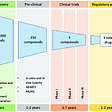## Shallow learnings on Deep Learning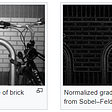## Evaluation Metrics for Textual Problems## Image Edge Detection using five lines of code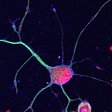## From the Bronze Age to Today’s AI-based Circular Economy, and How Data Virtualization and…## Convolutional Neural Network: A simple explanation## Summary of PixelRNN by Google Deepmind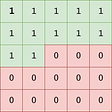http://dima.ai/

## Efficient Nets — Rethinking Model Scaling## 56: Safety Operating System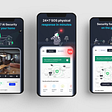## Image-Template matching using Cross-Correlation## Machine Learning in Storage Systems. Part 1